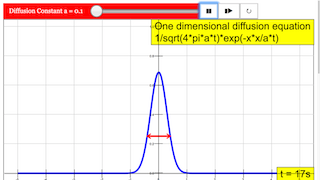Description

One dimensional diffusion equation

The simulation demonstrates the analytic solution of the one dimensional diffusion equation. A delta pulse at the origin is set as the initial function. This setup approximately models the temperature increase in a thin, long wire that is heated at the origin by a short laser pulse.

The analytic solution is a Gaussian spreading in time. Its integral is constant, which means that the laser pulse heating energy is conserved in the diffusion process.

To avoid the singularity of the delta function at time t = 0 the calculation starts at t = 0.0001 sec with a Gaussian of corresponding narrowness. It is visible as a blue line before start.

After Start the maximum amplitude (temperature) falls at a decreasing rate (observe the changing scale), while the width of the distribution grows correspondingly.

Arrows indicate the 1/e width. Time t is counted in an number field in seconds.

A slider defines the diffusion constant (heat conductivity) within a wide range.

Formula

One dimensional diffusion equation

∂Φ/∂t = a 2Φ/∂t2

With normalized delta function as initial function

Φ(0,0)  = δ(0) = 0 for x≠0       and δdx = 1

the analytic solution is a normalized Gaussian function.

Φ(x,t) = exp(-x2/at) / sqrt (4πat)

1/e- width:  at

maximum amplitude: 1 / sqrt (4πat)

Experiments

E1: Measure the time dependence of the maximum amplitude and draw its graph on log-linear paper. Choose an appropriate diffusion constant and consider the changing scale.

E2: Do the same for the 1/e width of the distribution. Use a log-quadratic system for drawing, too.

E3: Interpret your measurement by analysis of the Gaussian formula.

Translations

Code Language Translator RunSoftware Requirements

SoftwareRequirements

 Android iOS Windows MacOS with best with Chrome Chrome Chrome Chrome support full-screen? Yes. Chrome/Opera No. Firefox/ Samsung Internet Not yet Yes Yes cannot work on some mobile browser that don't understand JavaScript such as..... cannot work on Internet Explorer 9 and below

CreditsThis email address is being protected from spambots. You need JavaScript enabled to view it.; Fremont Teng; Loo Kang Wee

[text]

One Dimensional Diffusion Equation JavaScript Simulation Applet HTML5

Instructions

Diffusion Constant Slider

This will speed up the rate of diffusion over time under the below equation:

Toggling Full Screen

Double clicking anywhere in the panel will toggle full screen.
(Note that this won't work if the simulation is playing.)

Play/Pause, Step and Reset Buttons

Plays/Pauses, steps and resets the simulation respectively.

Research

[text]

[text]

[text]

Testimonials (0)

There are no testimonials available for viewing. Login to deploy the article and be the first to submit your review!